地球物理学报2015, Vol. 58Issue (12): 4661-4674PDF

1. 中南大学有色金属成矿预测与地质环境监测教育部重点实验室, 长沙 410083;
2. 中南大学地球科学与信息物理学院, 长沙 410083

Research on apparent resistivity and its influence of obliquely incident plane electromagnetic waves
XIAO Xiao1,2, FU Hong-Liu1,2, TANG Jing-Tian1,2, LIU Feng-Yi1,2, REN Zheng-Yong1,2, YUAN Yuan1,2, ZHANG Lin-Cheng1,2
1. Key Laboratory of Metallogenic Prediction of Non-ferrous Metals and Geological Environment Monitor Central South University, Ministry of Education, Changsha 410083, China;
2. Institute of Geosciences and Info-Physics, Central South University, Changsha 410083, China
Abstract: Both the conventional magnetotelluric method (MT) and audio-frequency magnetotelluric method (AMT) without considering the influence of displacement currents are based on the hypothesis that planar electromagnetic waves are normally incident on the surface of the earth. In reality, such a case is not true and in some cases, the displacement currents cannot be neglected. This paper analyzes the relative difference of apparent resistivity of TE and TM modes in the condition of considering the displacement currents and planar electromagnetic waves are obliquely incident on the surface of the earth.
The method that this paper adopted is numerical modeling. Based on Maxwell's equations, this paper deduces the impedance formula of planar electromagnetic waves that are obliquely incident on the layered earth under the total current condition in new ways and finishes the corresponding computation programs. The program correctness is verified with comparison to analytic solutions as well as published results. Based on this, the paper calculates and analyzes the apparent resistivity of TE and TM modes varying with displacement currents, incidence angle, magnetic permeability and dielectric permittivity under the quasi-static limit and the total current condition.
According to the various cases of the quasi-static limit and the total current condition, the following information of the relative difference of apparent resistivity of TE and TM modes could be obtained easily. 1) The change of the incidence angle has little impact on TE and TM modes' apparent resistivity under the quasi-static limit. In addition, the apparent resistivity has almost no difference between the two modes. 2) When the incidence angle equals zero, TE and TM modes' apparent resistivity equals each other, which is independent of earth resistivity, frequency, magnetic permeability and dielectric permittivity. As for earth resistivity is above 1000 Ωm, when the incidence angle is below 30 degrees and frequency is less than 1000 kHz, the difference of the apparent resistivity of normally incident and obliquely incident could be ignored. When the incidence angle is greater than 30 degrees and frequency is above 1000 kHz, the difference between the apparent resistivity of normally incident and obliquely incident could not be neglected. At the same time, TE-mode's resistivity increases with the increase of incidence angle, while for TM mode, the opposite holds. Therefore, the difference of the two modes' resistivity increases with increasing incidence angle. Which acts fairly significant especially when frequency is above 1000kHz and incidence angle equals 60 degrees. 3) When the plane electromagnetic wave normally incidents, whether it is under the quasi-static condition, or under the total currents condition, if half-space permeability is not equal to free-space permeability, the calculated apparent resistivity will be μr(μr, the relative permittivity) times of the true half-space resistivity. Under the total currents condition, if the plane electromagnetic wave obliquely incidents on the earth surface, TE-mode's resistivity will be a little smaller than μr times of the true half-space resistivity, while for TM mode, the opposite holds. This is not obvious when frequency is less than 100 kHz, while when frequency is greater than 100 kHz, it acts significantly. 4) As for earth resistivity is greater or equal to 2000 Ωm, the influence of dielectric permittivity on the differences of the two modes' resistivity could be ignored when frequency is less than 100 kHz. While when frequency is greater than 100 kHz, TE or TM-mode's resistivity decreases with the increase of dielectric permittivity. Under the condition of planar electromagnetic wave obliquely incidents, when there is a shallow overburden and frequency equals 1000 kHz, the relative error of the two modes' resistivity maintains 22.5% varying with dielectric permittivity.
In the exploration of the controlled-source audio-frequency magnetotelluric method (CSAMT), if the measured area is not far enough away from the source, it does not meet the planar wave hypothesis, hence the incidence angle should be taken into consideration. In AMT exploration, when the measurement point is located in a high-resistivity geological environment, the apparent resistivity curve of the high frequency section often drops compared to the simulation results under the quasi-static condition, probably because the displacement current cannot be ignored. In the actual exploration, the permeability of the shallow surface has a great influence on the high frequency apparent resistivity, especially when the measurement point is located in the ferromagnetic material. When at higher frequencies or high resistance region, the presence of the dielectric permittivity makes the apparent resistivity decrease. Therefore, in the actual data processing and inversion interpretation, for some special geological condition or at high frequency, the influences of incidence angle, displacement currents, magnetic permeability and dielectric permittivity should be taken into consideration, otherwise we cannot obtain the right conclusions.
Key words: Displacement currents     Incident angle     Magnetic permeability     Dielectric permittivity     Apparent resistivity     TE mode and TM mode
﻿1 引言

2 全电流时倾斜入射平面电磁波的阻抗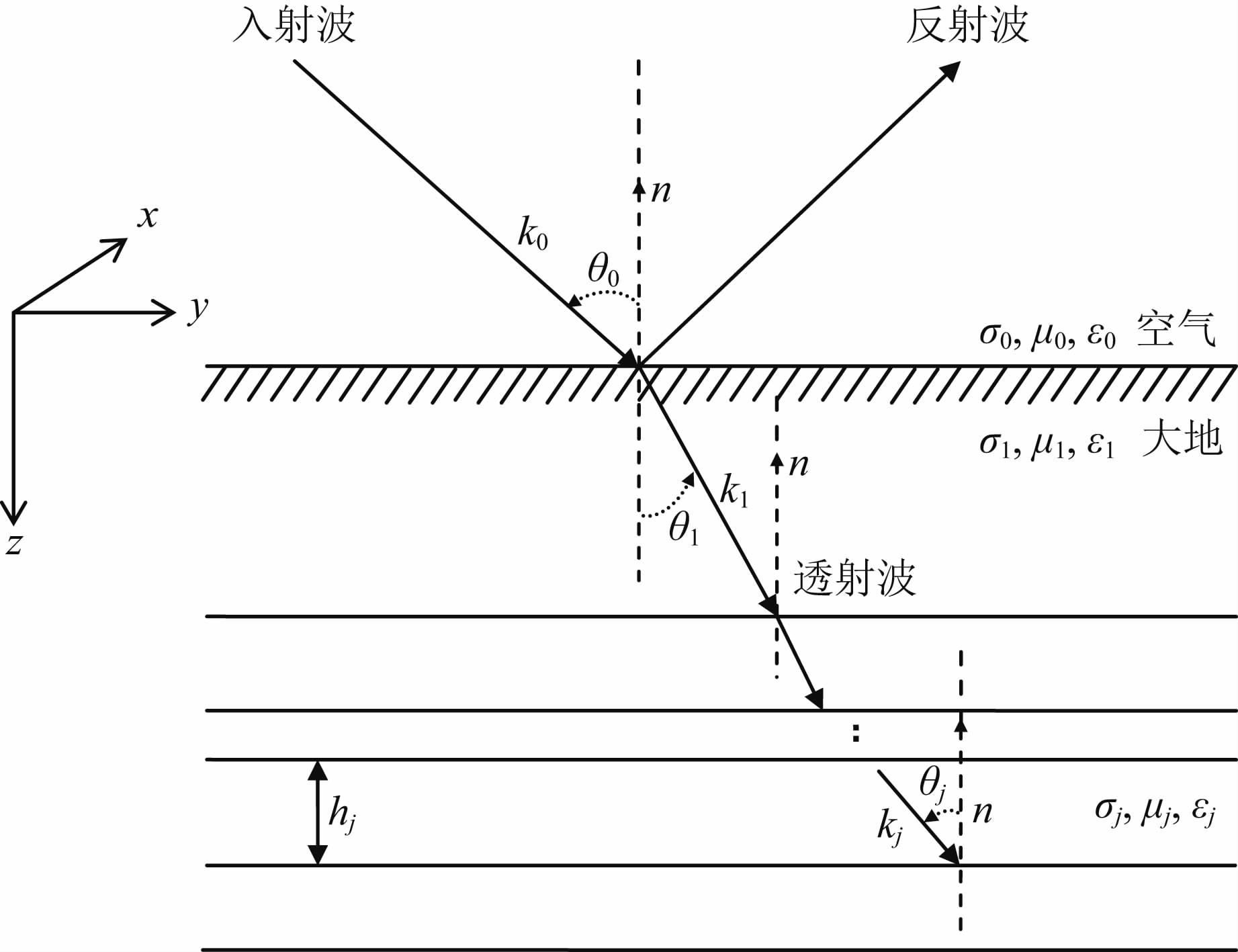图 1 平面波传播示意图 Fig. 1 Schematic diagram of plane wave propagation

(1)TE-mode：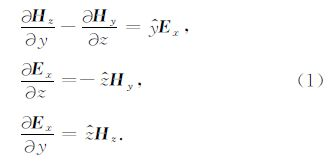(2)TM-mode：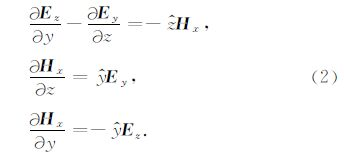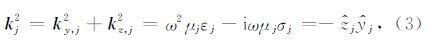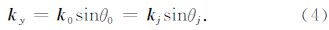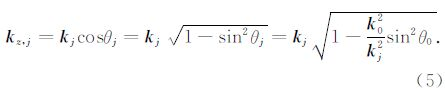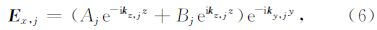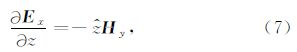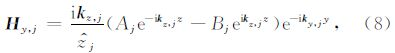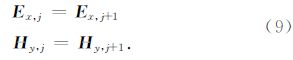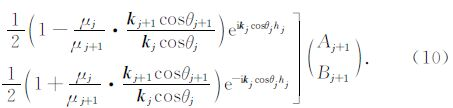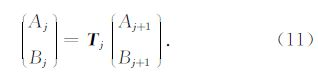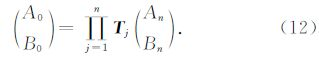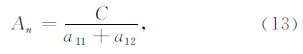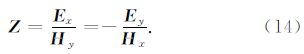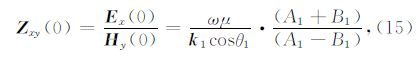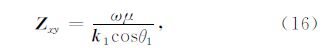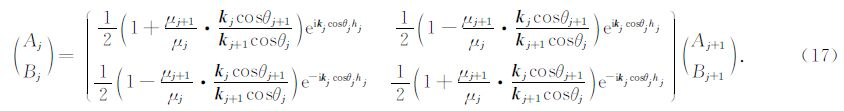n层地表波阻抗为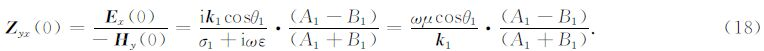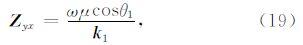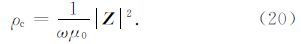3 计算程序验证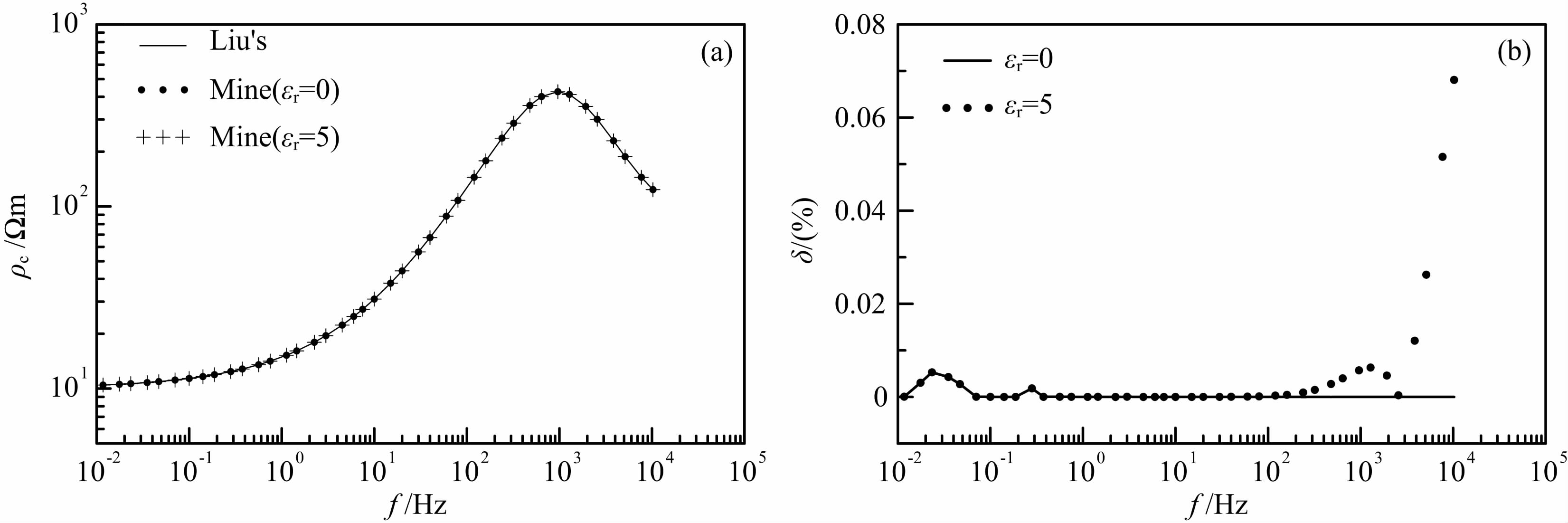图 2 三层模型上计算结果对比 (a)视电阻率曲线；(b)误差曲线.Liu′s曲线是刘长生(2009)给出的结果，Mine曲线是本文的结果.δ为相对误差. Fig. 2 Result comparison of three-layer mode (a)Apparent resistivity curves;(b)Relative error curves. Liu′s curve is the results given by Liu(2009); Mine curve is the results given by this study.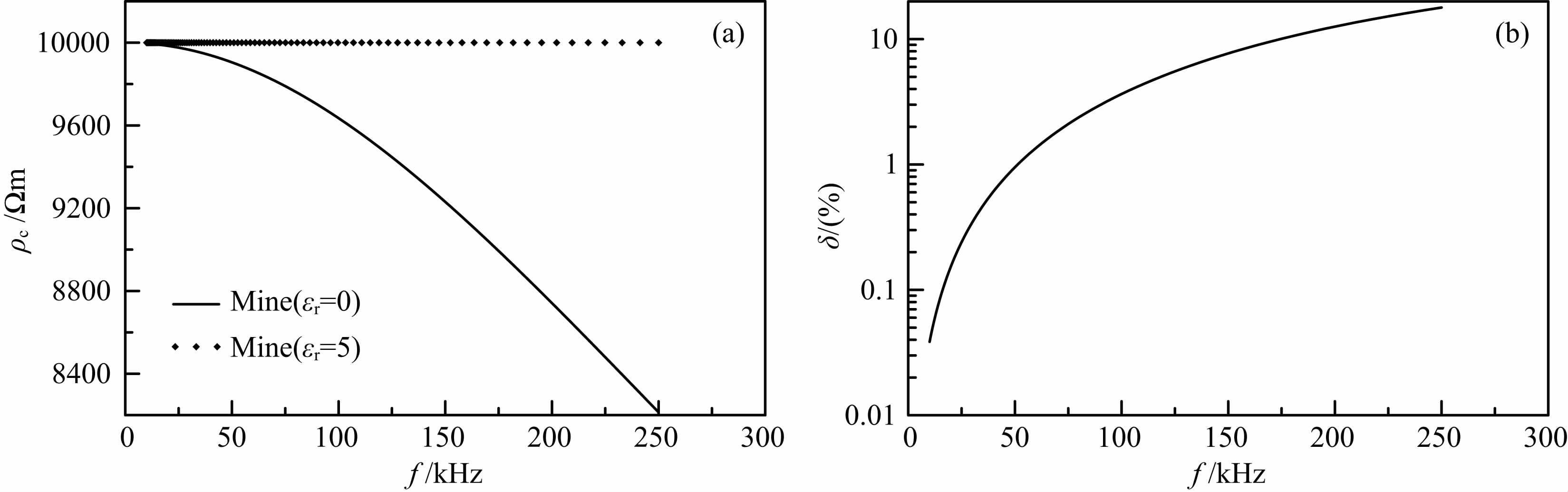图 3 均匀半空间模型上计算结果对比(a)视电阻率曲线；(b)误差曲线. Fig. 3 Result comparison of homogeneous mode(a)Apparent resistivity curves;(b)Relative error curves.
4 计算结果分析

4.1 准静态极限下的结果分析

4.1.1 入射角变化对TE、TM模式视电阻率的影响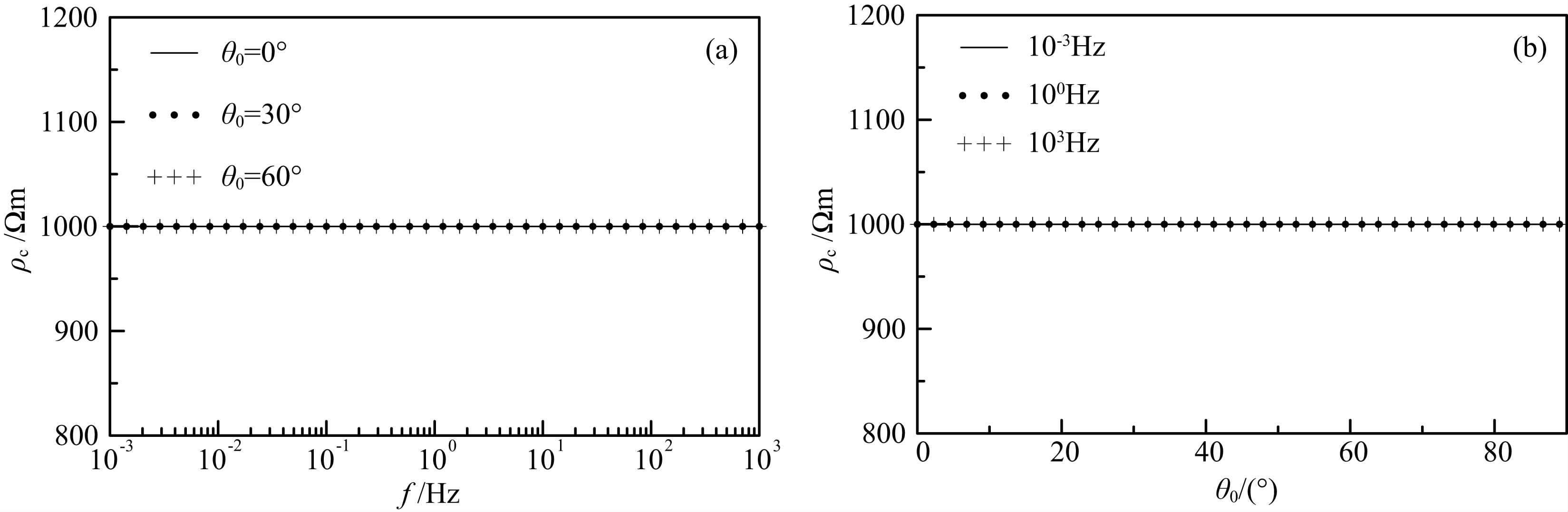图 4 均匀半空间(ρ=1000 Ωm)视电阻率曲线 (a)频率测深曲线;(b)电阻率随入射角变化曲线. Fig. 4 Apparent resistivity curves of homogeneous half-space(a)Frequency sounding curves;(b)Resistivity curves varied with incident angle.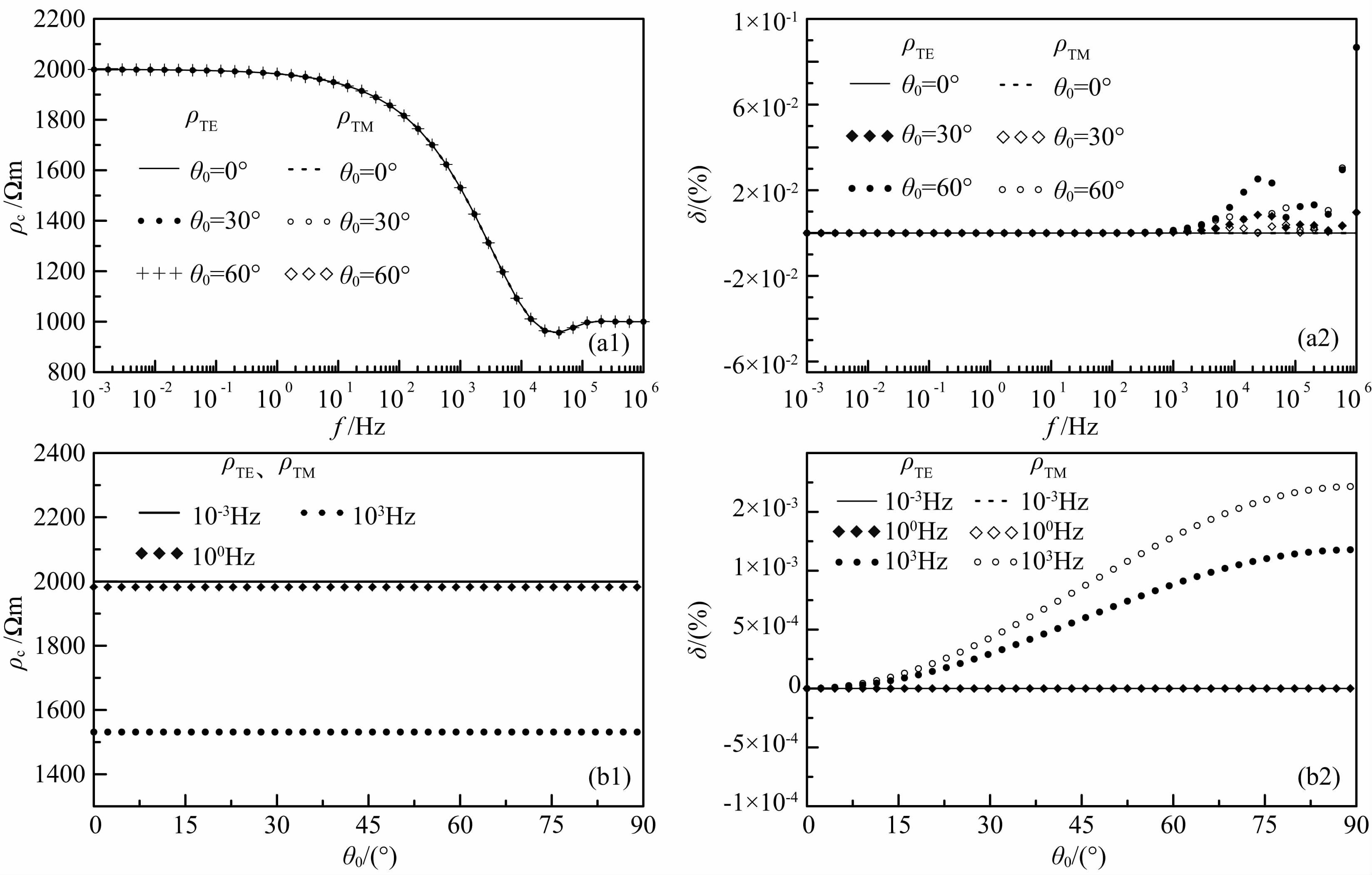图 5 二层介质(ρ1=1000 Ωm，h1=100 m；ρ2=2000 Ωm)视电阻率曲线及误差 (a1)频率测深曲线;(a2)误差曲线；(b1)电阻率随入射角变化曲线;(b2)误差曲线. Fig. 5 Apparent resistivity curves and errors of two-layer medium(a1)Frequency sounding curves;(a2)Corresponding relative error curves;(b1)Resistivity curves varied with incident angle; (b2)Corresponding relative error curves.

4.1.2 磁导率对视电阻率的影响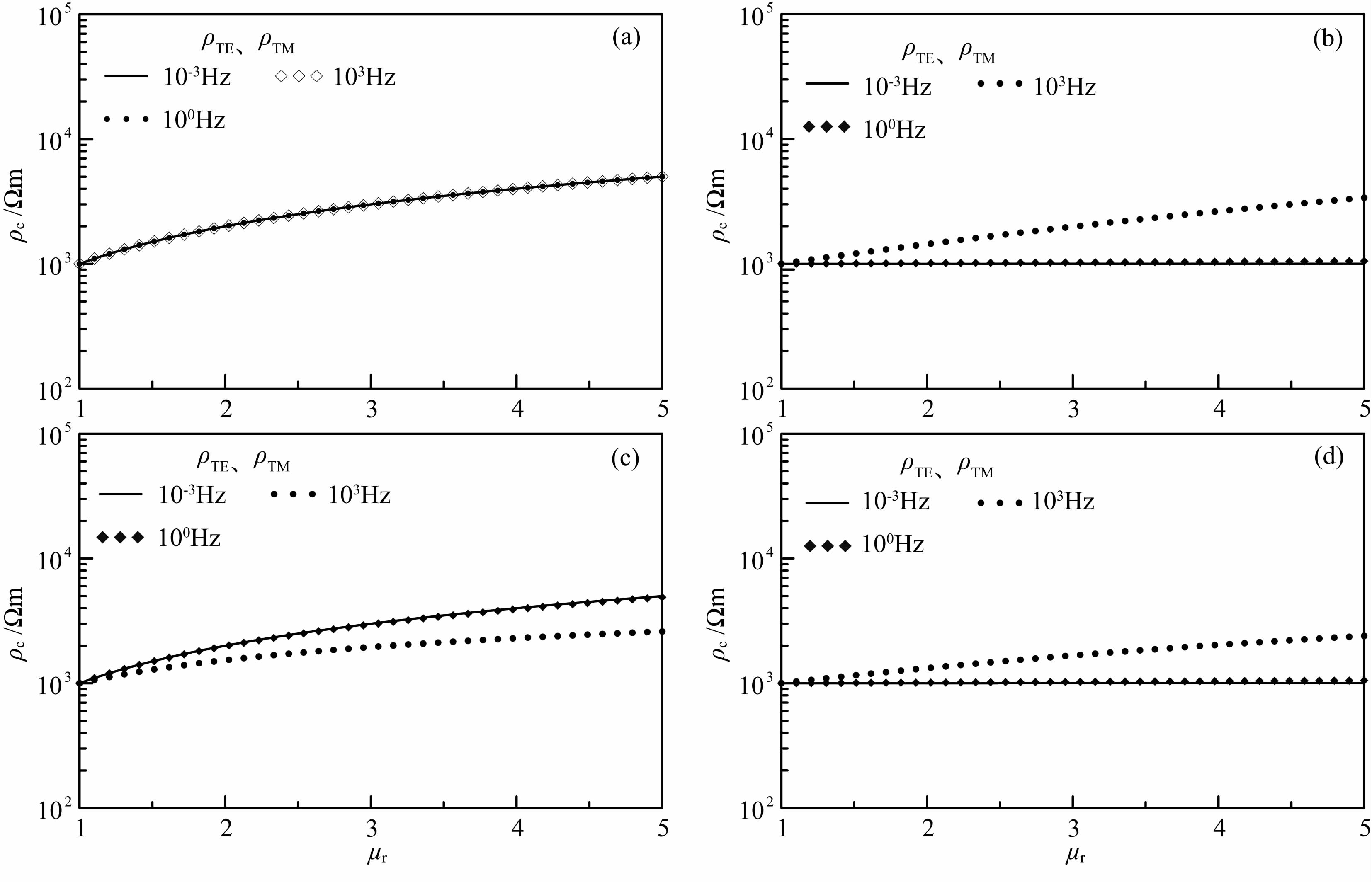图 6 不同频率下TE、TM模式视电阻率随磁导率的变化(a)均匀半空间(ρ=1000 Ωm)；(b)、(c)两层介质(ρ1=1000 Ωm，h1=100 m； ρ2=1000 Ωm)；(d)三层介质(ρ1=1000 Ωm，h1=100 m；ρ2=1000 Ωm，h2=100 m；ρ3=1000 Ωm). 其中入射角为θ0=0°，该情况下ρTE=ρTM. Fig. 6 The apparent resistivity of TE or TM mode varies with different magnetic permeabilities under different frequency(a)Homogeneous half-space;(b)，(c)Two-layer medium;(d)Three-layer medium. Where the incident angle θ0=0°，under this condition ρTE=ρTM.

4.2 全电流条件

i4

4.2.1 入射角变化对TE、TM模式视电阻率的影响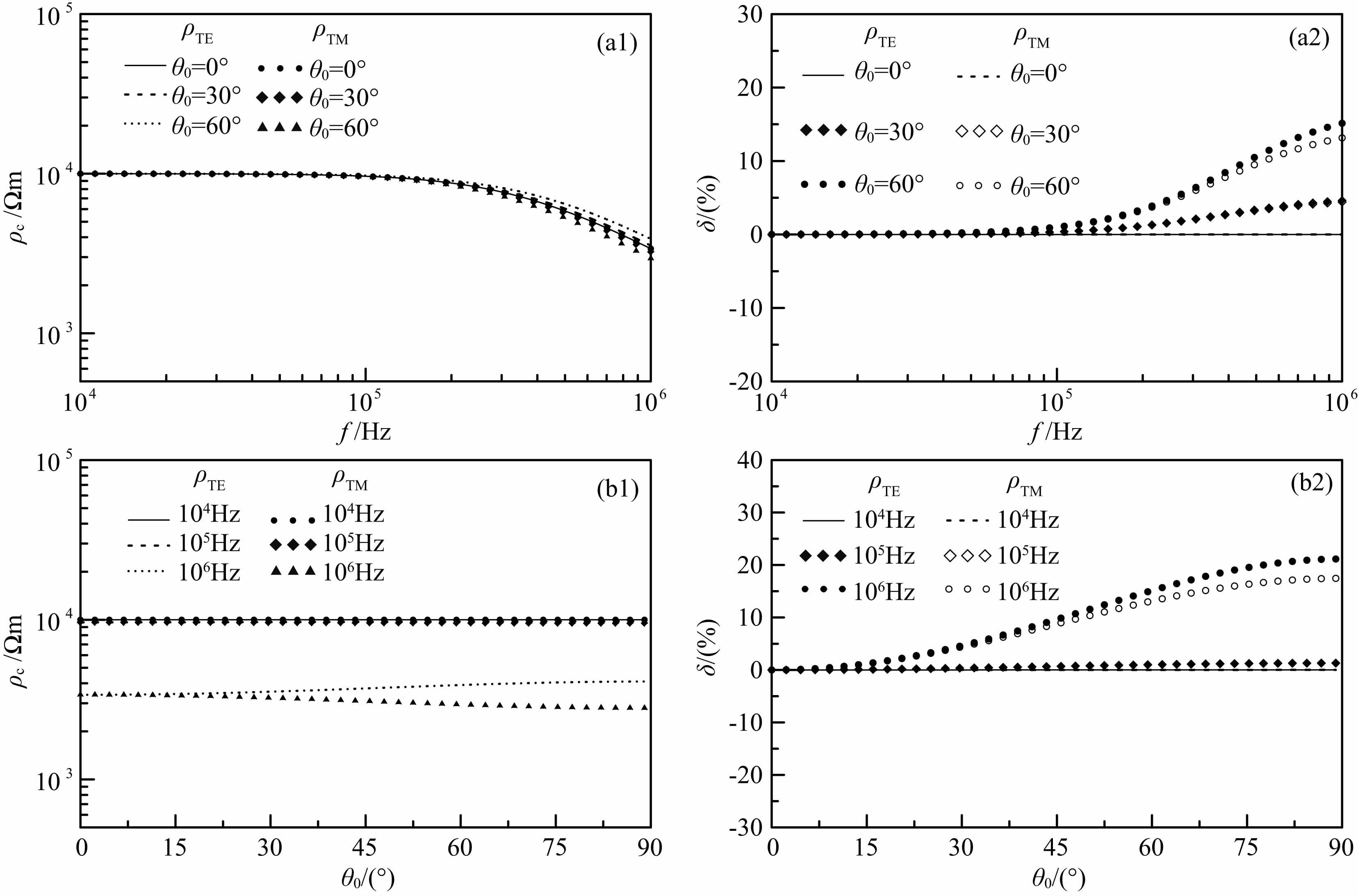图 7 均匀半空间(ρ=10000 Ωm)视电阻率曲线及误差(a1)频率测深曲线；(a2)误差曲线；(b1)电阻率随入射角变化曲线；(b2)误差曲线. Fig. 7 Apparent resistivity curves and errors of homogeneous half-space(a1)Frequency sounding curves;(a2)Corresponding relative error curves;(b1)Resistivity curves varied with incident angle; (b2)Corresponding relative error curves.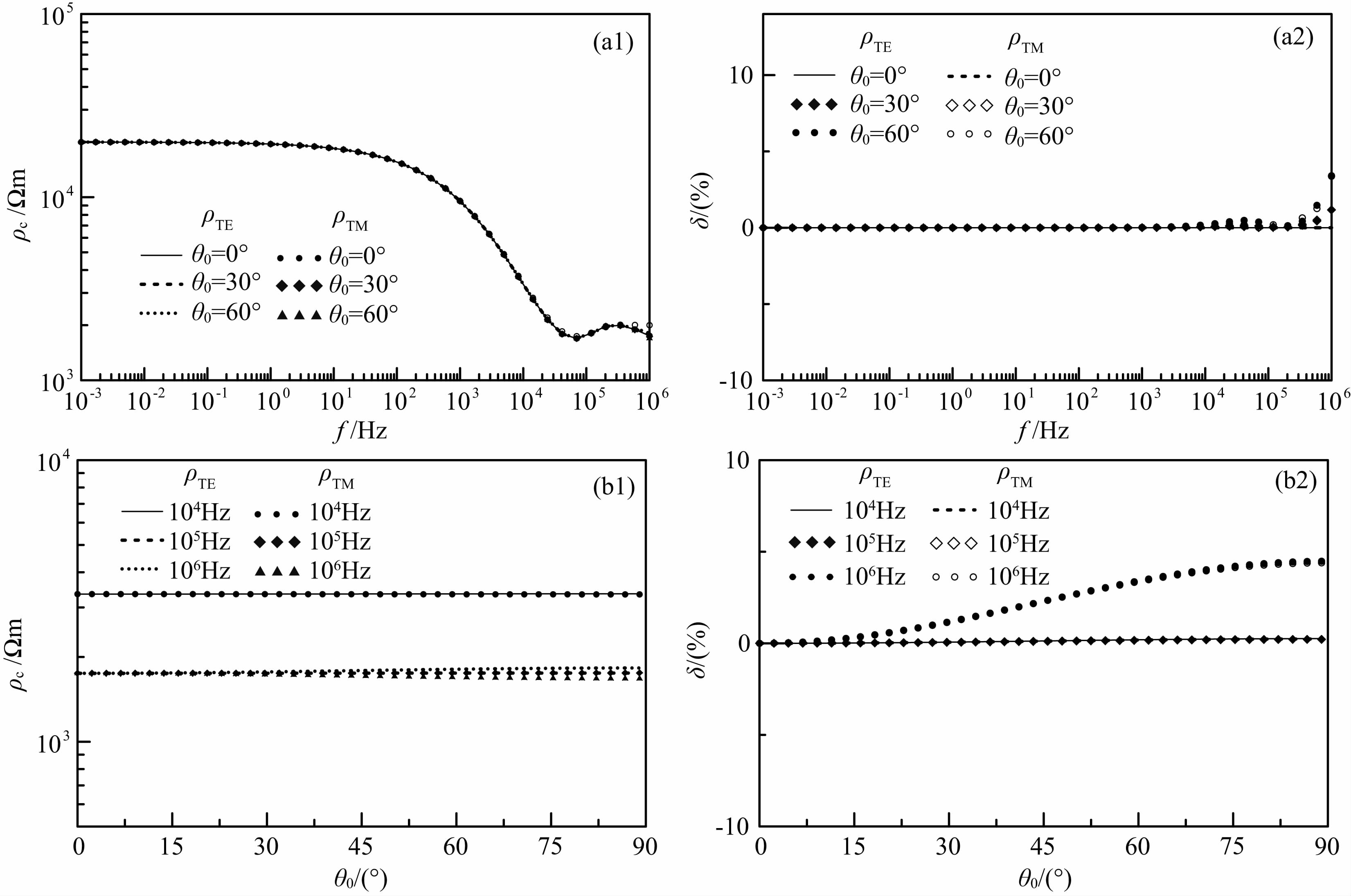图 8 两层介质(ρ1=2000 Ωm，h1=100 m；ρ2=20000 Ωm)视电阻率曲线及误差(a1)频率测深曲线;(a2)误差曲线;(b1)电阻率随入射角变化曲线;(b2)误差曲线. Fig. 8 Apparent resistivity curves and errors of two-layer medium(a1)Frequency sounding curves;(a2)Corresponding relative error curves;(b1)Resistivity curves varied with incident angle; (b2)Corresponding relative error curves.

4.2.2 磁导率变化对TE、TM模式视电阻率的影响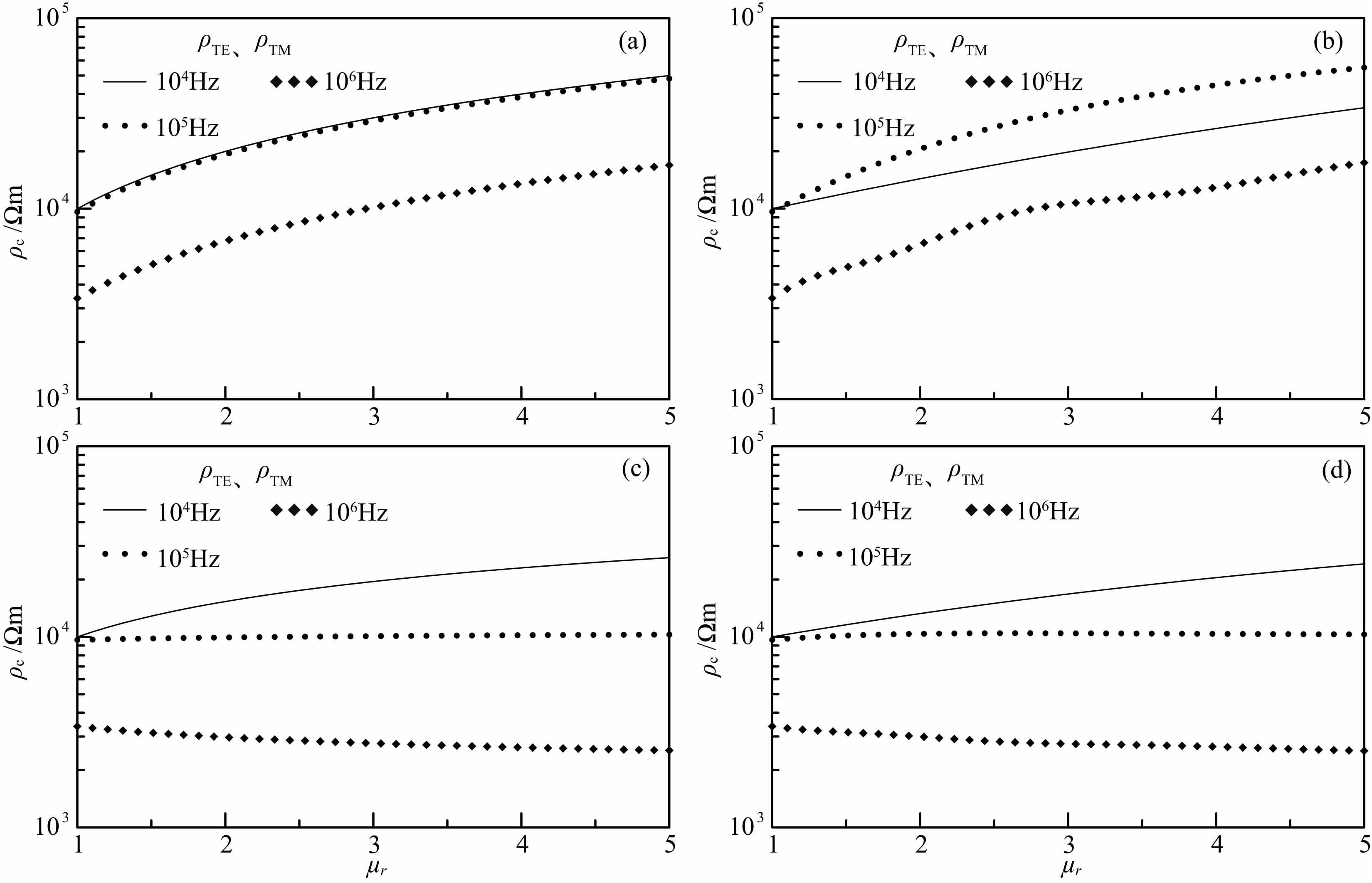图 9 不同磁导率下TE、TM模式视电阻率曲线(垂直入射) (a)均匀半空间；(b)、(c)两层介质；(d)三层介质. Fig. 9 Apparent resistivity curves of TE，TM mode among different magnetic permeabilities(normal incident)(a)Homogeneous half-space;(b)，(c)Two-layer medium;(d)Three-layer medium.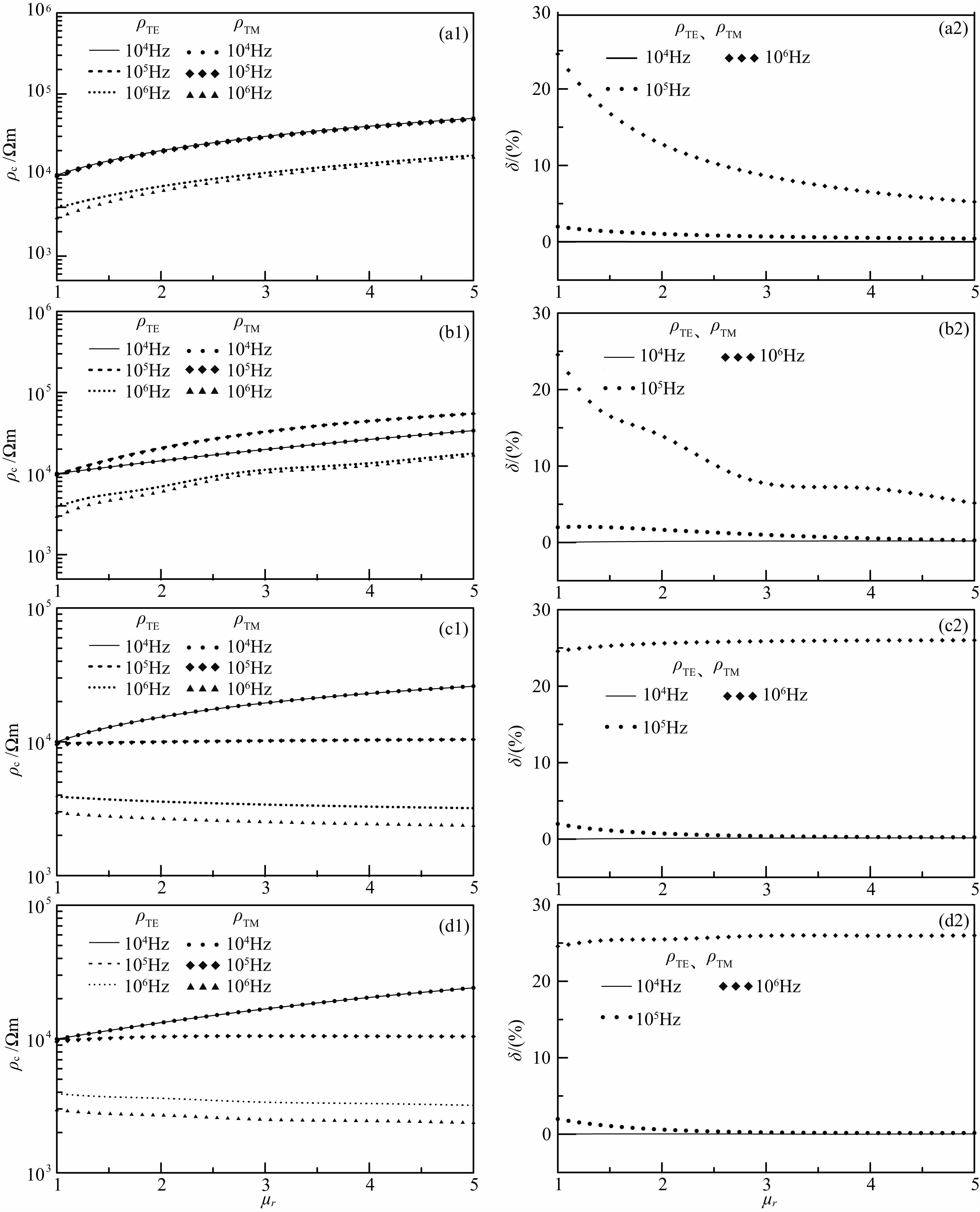图 10 不同磁导率下TE、TM模式视电阻率及其相对误差(倾斜入射)(a1)均匀半空间，(a2)相对误差；(b1)、(c1)两层介质，(b2)、(c2)相对误差；(d1)三层介质，(d2)相对误差. Fig. 10 Apparent resistivity curves of TE，TM mode among different magnetic permeabilities(obliquely incident)(a1)Homogeneous half-space;(a2)Corresponding relative error;(b1)，(c1)Two-layer medium; (b2)，(c2)Corresponding relative error;(d1)Three-layer medium，(d2)Corresponding relative error.

4.2.3 介电常数变化对TE、TM模式视电阻率的影响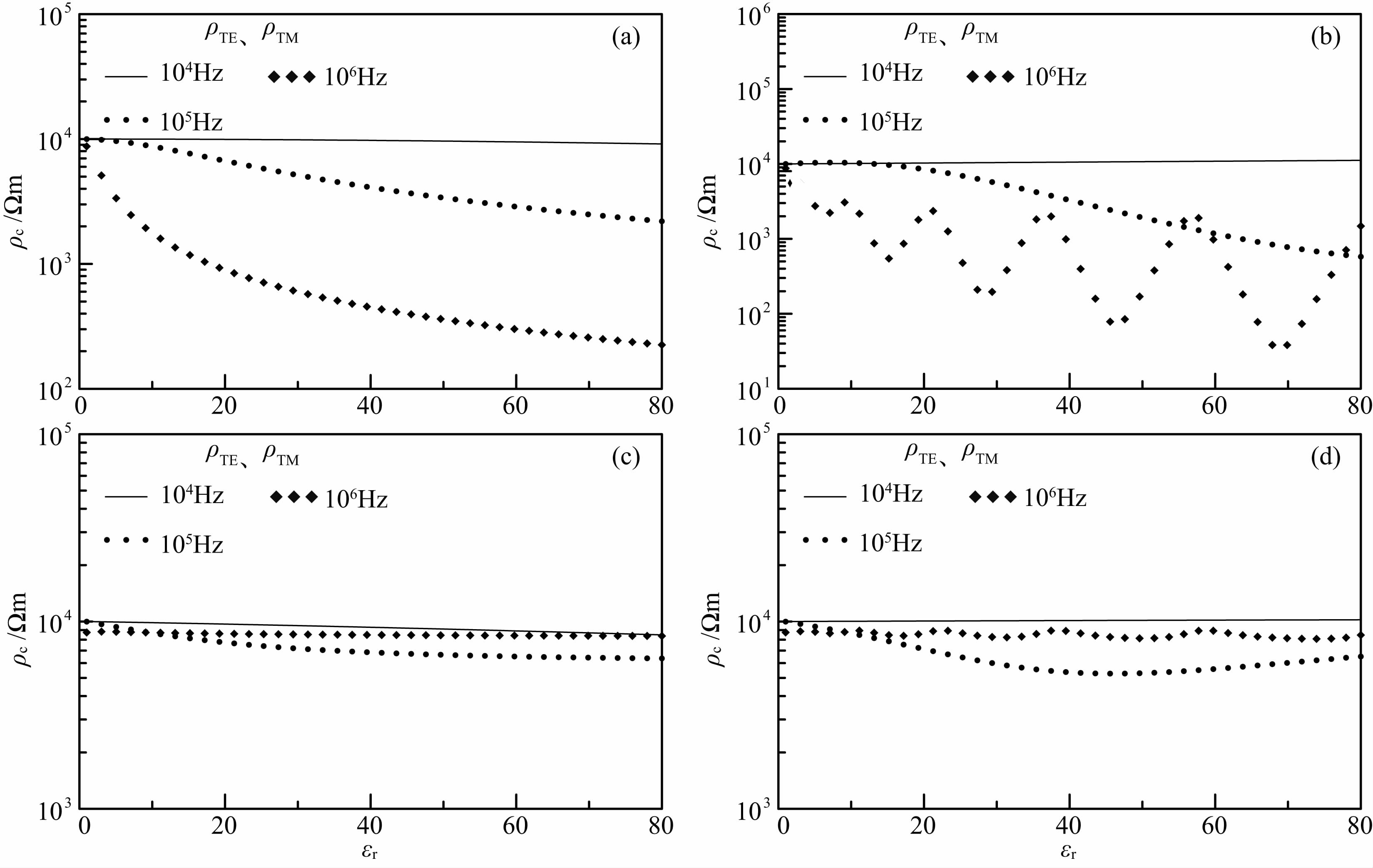图 11 不同介电常数下TE、TM模式视电阻率曲线(垂直入射) (a)均匀半空间；(b)、(c)两层介质；(d)三层介质. Fig. 11 Apparent resistivity curves of TE，TM mode among different dielectric permittivities(normal incident)(a)Homogeneous half-space;(b)，(c)Two-layer medium;(d)Three-layer medium.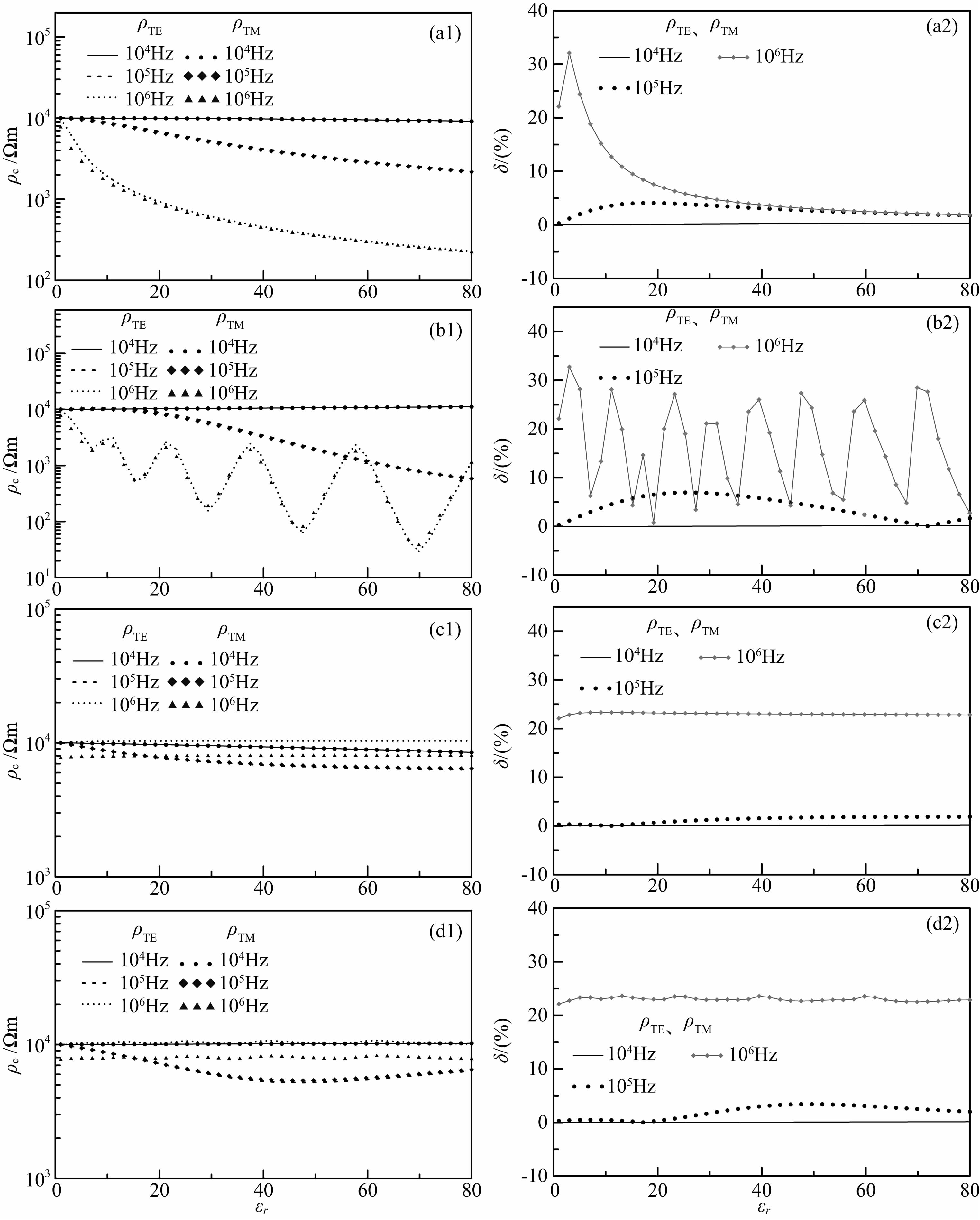图 12 不同介电常数下TE、TM模式视电阻率及其相对误差(倾斜入射) (a1)均匀半空间，(a2)相对误差；(b1)、(c1)两层介质，(b2)、(c2)相对误差；(d1)三层介质，(d2)相对误差. Fig. 12 Apparent resistivity curves of TE，TM mode among different dielectric permittivities(obliquely incident)(a1)Homogeneous half-space;(a2)Corresponding relative error;(b1)，(c1)Two-layer medium; (b2)，(c2)Corresponding relative error;(d1)Three-layer medium，(d2)Corresponding relative error.

5 结论

1)准静态极限条件下，入射角的变化(θ0≠90°)对层状介质表面ρTE和ρTM的影响可忽略不计，而且两种模式视电阻率之间几乎无差别.当入射角θ0=0°时，ρTE=ρTM，该特性与大地电阻率、频率、磁导率以及介电常数变化无关.对大地电阻率ρ≥ 1000 Ωm 的介质，当入射角θ0<30°且频率f<106 Hz时，电磁波倾斜入射计算的地表视电阻率同垂直入射计算的视电阻率之间的差别可忽略不计；当入射角θ0>30°且频率f≥106时，电磁波倾斜入射计算的地表视电阻率同垂直入射计算的视电阻率之间的差别不可忽略.同时，TE模式视电阻率随入射角的增大而增大，而TM模式视电阻率呈现相反的规律.因此，在全电流条件下，两种模式之间视电阻率的差别随入射角的增大而增大.当频率f>106 Hz，θ0=60°时，两者之间的差异十分明显.

2)当电磁波垂直入射，无论是在准静态极限条件下，还是在全电流条件下，当半空间磁导率不等于自由空间磁导率时，计算的视电阻率将是半空间真电阻率的μr倍.而全电流条件下，当电磁波倾斜入射时，TE模式视电阻率将比半空间真电阻率的μr倍要小些，TM模式视电阻率则将比半空间真电阻率的μr倍要大些.其在频率f<105 Hz时，表现不明显；而当f=106 Hz时，表现较显著.浅表覆盖层的存在使得磁导率对浅部(高频)视电阻率的影响减弱，而对深部(低频)视电阻率几乎无影响.电磁波倾斜入射时，ρTEρTM，而当频率f<105 Hz时，磁导率变化对两种模式之间视电阻率差别的影响可忽略不计；当频率f=106 Hz且无覆盖层时，两种模式视电阻率之间的差别随磁导率的增大而逐渐减小.当存在浅表覆盖层且频率f=106 Hz时，两种模式视电阻率之间的相对误差几乎保持在25%.

3)大地电阻率ρ≥2000 Ωm的高阻介质，当频率f<105 Hz，介电常数对ρTEρTM之间差别的影响可忽略不计.当频率f>105 Hz时，ρTEρTM随介电常数的增加而减小.覆盖层的存在使得介电常数的影响降低.电磁波倾斜入射时，当存在浅表覆盖层且频率f=106 Hz时，两种模式视电阻率之间的相对误差保持在22.5%左右.

4)CSAMT法勘探中，若测区离发射场源不是足够远，则其不满足平面电磁波垂直入射的假设，而需考虑入射角的影响.ATM勘探时，当测点位于高阻地质环境中，高频段的视电阻率曲线相比于准静态条件下的模拟结果常出现下掉现象，这很可能是因为位移电流不可忽略而引起.在实际勘探中，浅表层的磁导率对高频视电阻率有较大的影响，尤其是测点恰好位于铁磁性物质上，影响将会更大.在高阻区或频率较高时，介电常数的存在使得实际视电阻率数值降低.

5)由以上分析可知，实际数据处理及反演解释中，对特殊地质条件或频率很高时，应考虑位移电流、入射角、磁导率以及介电常数对TE和TM两种视电阻率模式之间差异的影响，否则可能得不到正确的结论.

  Fraser D C, Stodt J A, Ward S H. 1990. The effect of displacement currents on the response of a high-frequency electromagnetic system. Environmental and Groundwater.Soc.Expl.Geophysics,89-95.  He W G, Yan J B, Li J J. 2013. The influence of displacement current as well as reflection and refraction on the depth detection of high frequency electromagnetic wave. Chinese Journal of Engineering Geophysics (in Chinese), 10(4): 539-544.  Huang H P, Fraser D C. 2002. Dielectric permittivity and resistivity mapping using high-frequency, helicopter-borne EM data. Geophysics, 67(3): 727-738.  Huang W B. 2009. Research of magnetic parameters effect in the magnetotelluric sounding[Ph. D. thesis] (in Chinese). Chengdu: Chengdu University of Technology.  Kalscheuer T, Pedersen L B, Siripunvaraporn W. 2008. Radiomagnetotelluric two-dimensional forward and inverse modelling accounting for displacement currents. Geophys. J. Int., 175(2): 486-514.  Kaufman A A, Keller G V. 1987. Magnetotelluric Sounding Method (in Chinese). Liu G D, et al., Trans. Beijing: Seismological Press, 43-117.  Li J M. 2005. Electricfield and Electrical Prospecting (in Chinese). Beijing: Geological Publishing House.  Liu C S. 2009. Three-dimension magnetotellurics adaptive edge finite-element numerical simulation based on unstructured meshes[Ph. D. thesis] (in Chinese). Changsha: Central South University, 26-33.  Lin J. 2000. Trend of electromagnetic instrumentation for engineering and environment. Geophysical and Geochemical Exploration (in Chinese), 24(3): 167-177.  Nabighian M N. 1992. Electromagnetic Methods in Applied Geophysics-(The first volume)-Theory (in Chinese). Zhao J X, Wang Y J Trans. Beijing: Geological Publishing House, 172-189.  Доброхотва И А, Юдин М Н, Kou S W. 1983. The effect of permeability on the work achievements of magnetotelluric sounding method (magnetotelluric field method). Foreign Geological Exploration Technology (in Chinese), (8): 37-40.  Pavlov D. 2000. Abnormal conductivity and abnormal permeability effects in the time-domain electromagnetic data. Peng Q X Trans. Foreign Oil and Gas Exploration (in Chinese), 12(1): 119-123.  Poikonen A, Suppala I. 1989. On modeling airborne very low-frequency measurements. Geophysics, 54(12): 1596-1606.  Sinha A K. 1997. Influence of altitude and displacement currents on plane-wave EM fields. Geophysics, 42(1): 77-91.  Song Y, Kim H J, Lee K H. 2002. High-frequency electromagnetic impedance method for subsurface imaging. Geophysics, 67(2): 501-510.  Stewart D C, Anderson W L, Grover T P, et al. 1994. Shallow subsurface mapping by electromagnetic sounding in the 300 KHz to 30 MHz range: Model studies and prototype system assessment. Geophysics, 59(8): 1201-1210.  Tang J T, Wang Y, Du H K, et al. 2009. A study of high frequency magnetotelluric numerical modeling by finite element method. Computing Techniques for Geophysical and Geochemical Exploration (in Chinese), 31(4): 297-302.  Wang M Y, Di Q Y, Xu K, et al. 1971. Simultaneously reconstructed the images of three electrical parameters: permittivity, permeability and resistivity under 1-D condition using CSAMT data.// Chinese Meteorological Society. To celebrate the eighty birthday of Professor Guo Zong fen and the theoretical and applied geophysics (in Chinese). Beijing: Chinese Meteorological Society, 216-224.  Ward S H. 1971. Foreword and introduction. Geophysics, 36(1): 1-8.  Xi J J. 2010. A study of forward and inversion for frequency-domain airborne electromagnetic method in terms of permeability and resistivity[Ph. D. thesis] (in Chinese). Changchun: Jilin University.  Yin C C, Hodges G. 2005. Influence of displacement currents on the response of helicopter electromagnetic systems. Geophysics, 70(4): G95-G100.  Zheng S T, Zeng Z F, Wang Z J. 2006. High-frequency electromagnetic response of layer model and the influences of displacement currents. Journal of Jilin University (Earth Science Edition) (in Chinese), 36(Supplementary): 172-176.  Zheng S T. 2007. High frequency electromagnetic modeling and inversion[Ph. D. thesis] (in Chinese). Changchun: Jilin University.  Zhu K G, Lin J, Cheng D F. 2002. Numerical computation of high frequency electromagnetic fields under MATLAB. Chinese Journal of Radio Science (in Chinese), 17(3): 224-228.  Zhu K G, Lin J, Ling Z B, et al. 2002. Study on investigation depth under high frequency electromagnetic fields caused by vertical magnetic dipole. Journal of Jilin University (Earth Science Edition) (in Chinese), 32(4): 390-393.  贺文根, 严家斌, 李俊杰. 2013. 位移电流及反射与折射对高频电磁波探测深度的影响. 工程地球物理学报, 10(4): 539-544.  黄文彬. 2009. 大地电磁测深中磁参数的影响研究[博士论文]. 成都: 成都理工大学.  考夫曼 A A, 凯勒 G V. 1987. 大地电磁测深法. 刘国栋等译. 北京: 地震出版社, 43-117.  李金铭. 2005. 地电场与电法勘探. 北京: 地质出版社.  刘长生. 2009. 基于非结构化网格的三维大地电磁自适应矢量有限元数值模拟[博士论文]. 长沙: 中南大学, 26-33.  林君. 2000. 电磁探测技术在工程与环境中的应用现状. 物探与化探, 24(3): 167-177.  Nabighian M N. 1992. 勘查地球物理电磁法-(第一卷)—理论. 赵经祥,王艳君译. 北京: 地质出版社, 172-189.  Доброхотва И А, Юдин М Н, 寇绳武. 1983. 磁导率对大地电磁测深法(磁大地电流场法)工作成果的影响. 国外地质勘探技术, (8): 37-40.  Pavlov D. 2000. 时间域电磁资料中的异常电导率和异常磁导率效应. 彭清香 译. 国外油气勘探, 12(1): 119-123.  汤井田, 王烨, 杜华坤等. 2009. 高频大地电磁法有限元数值模拟. 物探与化探计算技术, 31(4): 297-302.  王妙月, 底青云, 许琨等. 2002. 利用CSAMT资料同时重构1-D介电常数、导磁率和电阻率三个电性参数的像.// 中国气象学会. "庆贺郭宗汾教授八十寿辰"暨理论与应用地球物理研讨会论文集. 北京: 中国气象学会: 216-224.  习建军. 2010. 基于磁导率和电阻率的频率域航空电磁法正反演研究[博士论文]. 长春: 吉林大学.  郑圣谈, 曾昭发, 王者江. 2006. 层状介质高频电磁场响应及位移电流影响. 吉林大学学报( 地球科学版), 36(增刊): 172-176.  郑圣谈. 2007. 高频电磁场模拟及其反演[博士论文]. 长春: 吉林大学.  朱凯光, 林君, 程德福. 2002a. 基于Matlab的高频谐变电磁场的数值计算. 电波科学学报, 17(3): 224-228.  朱凯光, 林君, 凌振宝等. 2002b. 高频垂直磁偶极子电磁场勘探深度的研究. 吉林大学学报(地球科学版), 32(4): 390-393.